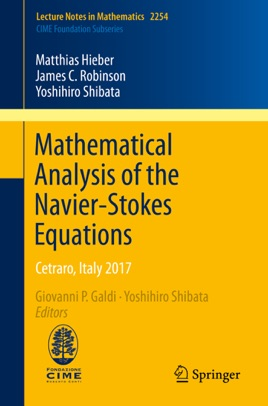• 37,99 €

## Description de l’éditeur

This book collects together a unique set of articles dedicated to several fundamental aspects of the Navier–Stokes equations. As is well known, understanding the mathematical properties of these equations, along with their physical interpretation, constitutes one of the most challenging questions of applied mathematics. Indeed, the Navier–Stokes equations feature among the Clay Mathematics Institute's seven Millennium Prize Problems (existence of global in time, regular solutions corresponding to initial data of unrestricted magnitude).

The text comprises three extensive contributions covering the following topics: (1) Operator-Valued H∞-calculus, R-boundedness, Fourier multipliers and maximal Lp-regularity theory for a large, abstract class of quasi-linear evolution problems with applications to Navier–Stokes equations and other fluid model equations; (2)  Classical existence, uniqueness and regularity theorems of solutions to the Navier–Stokes initial-value problem, along with space-time partial regularity and investigation of the smoothness of the Lagrangean flow map; and (3) A complete mathematical theory of R-boundedness and maximal regularity with applications to free boundary problems for the Navier–Stokes equations with and without surface tension.

Offering a general mathematical framework that could be used to study fluid problems and, more generally, a wide class of abstract evolution equations, this volume is aimed at graduate students and researchers who want to become acquainted with fundamental problems related to the Navier–Stokes equations.